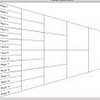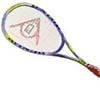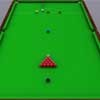You may also likeKnock-out

Before a knockout tournament with 2^n players I pick two players. What is the probability that they have to play against each other at some point in the tournament?Squash

If the score is 8-8 do I have more chance of winning if the winner is the first to reach 9 points or the first to reach 10 points?Snooker

A player has probability 0.4 of winning a single game. What is his probability of winning a 'best of 15 games' tournament?

Dicey Decisions

Age 16 to 18Challenge Level

Daniel from Savile Park School sent us a solution to the first part of the problem.

Here's a list of all the possible scores and how many possibilities they are for each score when an edge faces upwards:

 Score Combination 3 (1,2) 4 (1,3) 5 (1,4), (2,3) 6 (1,5), (2,4) 8 (2,6), (3,5) 9 (3,6), (4,5) 10 (4,6) 11 (5,6)

and another table for the possible scores and combinations for ending up a corner facing upwards:

 Score Combination 6 (1,2,3) 7 (1,2,4) 9 (1,3,5) 10 (1,4,5) 11 (2,3,6) 12 (2,4,6) 14 (3,5,6) 15 (4,5,6)

In the first case where the probabilities of landing with a face, edge or corner facing upwards are $\frac{1}{2}, \frac{1}{3}$ and $\frac{1}{6}$ respectively, the total probabilities of getting a number are shown in this table:

 Score Probability 144$\times$ probability 1 1/12 12 2 1/12 12 3 1/9 16 4 1/9 16 5 5/36 20 6 23/144 23 7 1/48 3 8 1/18 8 9 11/144 11 10 7/144 7 11 7/144 7 12 1/48 3 14 1/48 3 15 1/48 3

We need to arrange the outcomes into sets so that the sum of the probabilities in each set are equal. For example, for 3 people, a possible arrangement is:

Person 1: scores of 1,6,7,10,12

Person 2: scores of 2,3,5

Person 3: scores of 4,8,9,11,14,15

These 3 sets all sum to $\frac{1}{3}$ as required. There are other solutions similar to this example as you can freely exchange scores 10 and 11, scores 3 and 4, scores 1 and 2 and scores 12,14 and 15, without changing the sums of the probabilities.

There are likely to be more solutions, have you found any others?

Meg, from All Hallows school, wrote to us with a solution for the second part.

When the dice lands on a face, edge or corner with probability p, q and r, the total probabilities for getting each score are:

 Score Probability 24$\times$ probability 1 p/6 4p 2 p/6 49 3 p/6 + q/12 4p+2q 4 p/6 + q/12 4p+2q 5 p/6  +2q/12 4p+4q 6 p/6+ 2q/12 + r/8 4p+4q+3r 7 r/8 3r 8 2q/12 4q 9 2q/12 + r/8 4q+3r 10 q/12 + r/8 2q+3r 11 q/12 + r/8 2q+3r 12 r/8 3r 14 r/8 3r 15 r/8 3r

If there are n members of the family, we need to split the outcomes into n sets, such that the sums of the probabilities in each set are constant (equal to 1/n) for all p,q and r.

This means we need to partition these numbers into sets so that the probabilities sum in each set  to give the same quantity of p, the same quantity of q and the same quantity of r. These sums of probabilities will therefore be the same, whatever the values p, q and r take.

The sums will only involve integer multiples of 4p, 2q and 3r. We need to evenly split 6 multiples of 4p, 12 multiples of 2q and 8 multiples of 3r among n family members. What restrictions does this place on n? We need n to divide 6, 12 and 8, which implies n=2.

One possible arrangement is:

1) 1,3,5,8,10,12,14

2) 2,4,6,7,9,11,15

These sets both add up to 1/2. Again, there's degeneracy due to being able to interchange scores with the same probability.

Symmetry of probabilities:

The probabilities for the scores are symmetric because a cube, and therefore the set of possible scores, is unchanged if you interchange 1 and 6, 2 and 5, 3 and 4.